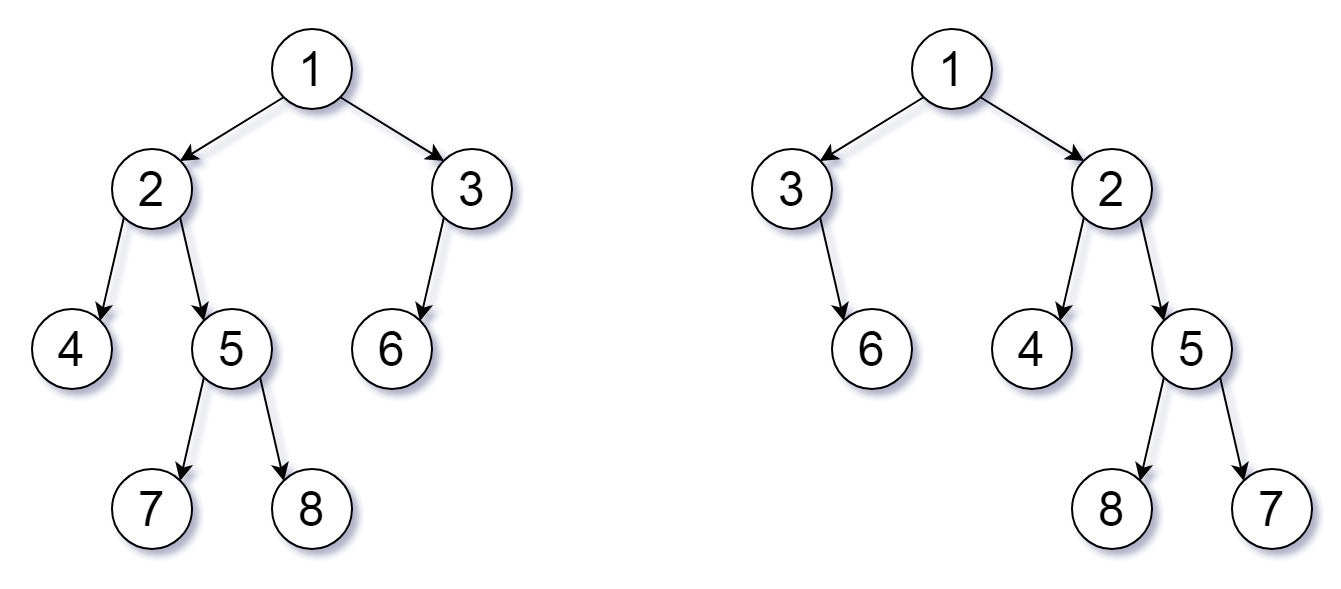Formatted question description: https://leetcode.ca/all/951.html

# 951. Flip Equivalent Binary Trees (Medium)

For a binary tree T, we can define a flip operation as follows: choose any node, and swap the left and right child subtrees.

A binary tree X is flip equivalent to a binary tree Y if and only if we can make X equal to Y after some number of flip operations.

Write a function that determines whether two binary trees are flip equivalent.  The trees are given by root nodes root1 and root2.

Example 1:

Input: root1 = [1,2,3,4,5,6,null,null,null,7,8], root2 = [1,3,2,null,6,4,5,null,null,null,null,8,7]
Output: true
Explanation: We flipped at nodes with values 1, 3, and 5.Note:

1. Each tree will have at most 100 nodes.
2. Each value in each tree will be a unique integer in the range [0, 99].

Related Topics:
Tree

## Solution 1.

// OJ: https://leetcode.com/problems/flip-equivalent-binary-trees/

// Time: O(N)
// Space: O(logN)
class Solution {
public:
bool flipEquiv(TreeNode* root1, TreeNode* root2) {
if (!root1 && !root2) return true;
if (!root1 || !root2) return false;
if (root1->val != root2->val) return false;
return (flipEquiv(root1->left, root2->left) && flipEquiv(root1->right, root2->right))
|| (flipEquiv(root1->left, root2->right) && flipEquiv(root1->right, root2->left));
}
};


Java

/**
* Definition for a binary tree node.
* public class TreeNode {
*     int val;
*     TreeNode left;
*     TreeNode right;
*     TreeNode(int x) { val = x; }
* }
*/
class Solution {
public boolean flipEquiv(TreeNode root1, TreeNode root2) {
if (root1 == null && root2 == null)
return true;
if (root1 == null ^ root2 == null)
return false;
queue1.offer(root1);
queue2.offer(root2);
while (!queue1.isEmpty()) {
if (queue2.isEmpty())
return false;
TreeNode node1 = queue1.poll(), node2 = queue2.poll();
if (node1.val != node2.val)
return false;
TreeNode left1 = node1.left, right1 = node1.right, left2 = node2.left, right2 = node2.right;
if (left1 == null && right1 == null) {
if (left2 != null || right2 != null)
return false;
} else {
if (left1 == null) {
left1 = right1;
right1 = null;
}
if (left2 == null) {
left2 = right2;
right2 = null;
}
if (left1 == null ^ left2 == null)
return false;
if (right1 == null ^ right2 == null)
return false;
if (right1 == null && left1.val != left2.val)
return false;
if (left1 != null && right1 != null) {
if (left1.val == right2.val) {
TreeNode temp = left2;
left2 = right2;
right2 = temp;
}
}
if (left1 != null)
queue1.offer(left1);
if (right1 != null)
queue1.offer(right1);
if (left2 != null)
queue2.offer(left2);
if (right2 != null)
queue2.offer(right2);
}
}
return queue2.isEmpty();
}
}